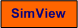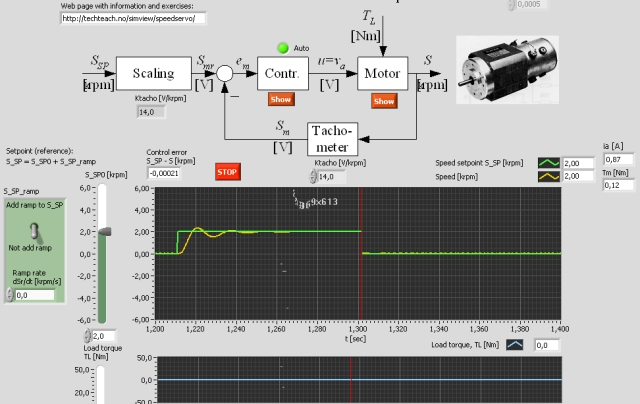# Speed Servo

Snapshot of parts of the front panel of the simulator:• What is needed to run the the simulator? Read to get most recent information!
• Tips for using the simulator.
• The simulator: . The simulator runs immediately after the download by clicking Open in the download window. Alternatively, you can first save a copy of the exe-file on any directory (folder) on your PC and then run the exe-file, which starts the simulator.

## Description of the simulated system

A speed control system - i.e. a speed servo -- is simulated. The motor is the DC motor Electro-Craft S-19-3AT which is an armature controlled, voltage controlled DC motor.

The speed is measured using a tachometer. The tachometer  gain and the other motor parameters can be adjusted from the front panel of the simulator.

## Mathematical model

In the expressions below the following quantities are used: S is rotational speed. va is armature voltage. ia is armature current. Tf  is friction torque. TL is load torque. J is the sum of the intertias of the motor and the load, i.e. J = Jmotor + Jload. B is the sum of the damping coefficients of motor and load. La is the armature inductance. Ra is the armature resistance. Ke is the voltage constant (the so called back-emf constant). KT is the torque constant.

The values of the motor parameters can be adjusted from the front panel.

### Dynamic model (differential equation)

A mathematical model of the motor can be found from the Kirchhoff's voltage law on the armature circuit and the torque balance of the mechanical rotation (refererence):

Kirchhoffs voltage law yields

(1) Ladia/dt = va - Raia - KeS

Torque balance yields

(2) JdS/dt = KTia - BS - Tf - TL

### Transfer function model

By taking the Laplace transform of the model above we will find the following transfer function from armature voltage va to speed S:

(3) S(s)/va(s) = H(s) = K/[(s/w0)2 + 2z(s/w0) + 1]

where the parameters are as follows:

Gain:

(4) K = Kt/(KtKe+BRa) [rpm/V]

Relative damping factor:

(5) z = 0.5*(JRa+BLa)/[sqrt(JLa(KtKe+BRa))]

Undamped resonance frequency:

### Static model

By setting the derivatives in (1) and (2) equal to zero and eliminating ia, we get the following static model (all variables are assumed to have constant values):

(7) S = [Kt/(KtKe+BRa)]va - [Ra/(KtKe+BRa)](Tf + TL)

## Aim

The aim of this simulator is to get a better understanding of how a speed control system works dynamically.

## Motivation

DC motors are used in many servo systems. Compared to other electro motors DC motors are easy to model mathematically, and, hence, easy to simulate.

In the tasks below is assumed - unless otherwise stated - that the process (motor) is in its normal or nominal operating point, defined as follows:

• The speed S is 3.0 krpm (revolutions per minute), which is used as a nominal speed setpoint.
• The load torque TL is 0 Nm.

Unless other information is given, it is assumed that the simulator runs while you do the tasks.

1. Control using constant control signal. Set the controller in manual mode.
1. Find the nominal control signal u0 experimentally (on the simulator) which brings the process to the nominal operating point.
2. Change the load torque (disturbance) from 0 to -20 Nm. What is the steady-state control error?

2. Tuning a PID control: In the subsequent tasks the motor speed is controlled by a PID controller (unless otherwise stated). Adjust the controller using the Ziegler-Nichols' closed loop method.

1. Let the the load torque be zero and the speed setpoint 3.0 krpm. What is the steady-state control error?
2. Change the load torque as a step frm 0 to 20 Nm. What is the steady state control error? Is there an improvement from task 1b?
3. Are the static setpoint tracking and disturbance tracking perfect?

4. Control using a P controller:
1. Find a proper value of the controller gain.
2. Are the static setpoint tracking and disturbance tracking perfect with a P controller? (Simulate!)

5. Tracking a setpoint ramp: Let the load torque be constant. Let the setpoint be a ramp of slope 100 krpm/s.
1. Use a PID controller. What is the steady state control error (as read off from the front panel of the simulator)?
2. Use a P controller. What is the steady state control error?

6. The stability of the control system at parameter changes: Let the setpoint be 3.0 krpm and the load torque 0 Nm. Observe what happen to the control system stability at the parameter changes described below. In each task/experimentyou can excite the control system via a small step in the setpoint. The experiments should be performed independently, i.e. the parameter values should be reset to default values once each of the experiments is finished.
1. The control gain is increased (much).
2. The integral time is decreased (much).
3. The derivative time is increased (much).
4. The tachometer gain (measurment gain) is increased  (much).
5. First, set the total damping coefficient B to zero. Then, reduce the intertia of the load (much). Try to explain the influence that this reduction has on the stability of the control system from Eq. 5.

[SimView] [TechTeach]

Updated 30. May 2008. Developed by Finn Haugen. E-mail: finn@techteach.no.